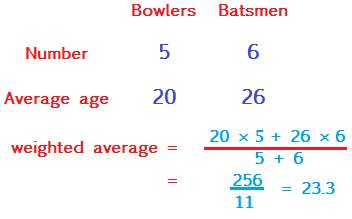## Weighted mean

Weighted average or weighted mean is the average of two or more groups.

Consider a cricket team of 11 players.

Divide them into two teams.

One team has 5 bowlers and another team has 6 batsmen.

Let the average age of the bowlers’ team be 20 years and that of the batsmen’ 26.

Now, what will be the average age of all the 11 players Or

What is the weighted mean age of the two teams combinedly?

The following table describes the data:The Weighted average of the two teams of bowlers and batsmen is 23.3 rounded to the tenth.

WEIGHTED AVERAGE PROPERTY

As a rule, the weighted mean is always closer to the average of the group that contains more number of terms.

This is what weight signifies in the name weighted average.

Weightage is greater weight resulting from greater number of terms.

Whichever group has greater number of terms carries greater weight.

And therefore, the weighted average leans to the average of this group.

In the above example, between the two averages 20 and 26, it is 26 to which the weighted average 23.3 is nearer to, because, 26 is the average age of the batsmen - the group that contains more number of terms.

Solve the following question using the above rule on weighted average:

In an institute having 20 employees of faculty and managers, there are 11 managers whose average salary is P. The average salary of the faculty, which is less than that of the others, is Q.

Is the average salary of all the 20 members in the office greater than
(P + Q)/2?

(P + Q)/2 is the mid-point of P and Q.

The number of managers is more than the number of faculty.

Therefore, weighted mean will lie closer to P than to Q.

Also, since P > Q, therefore weighted average lying closer to P will move beyond

(P + Q)/2.

Therefore, weighted average > (P + Q)/2

The following table will illustrate and clarify the weighted average rule